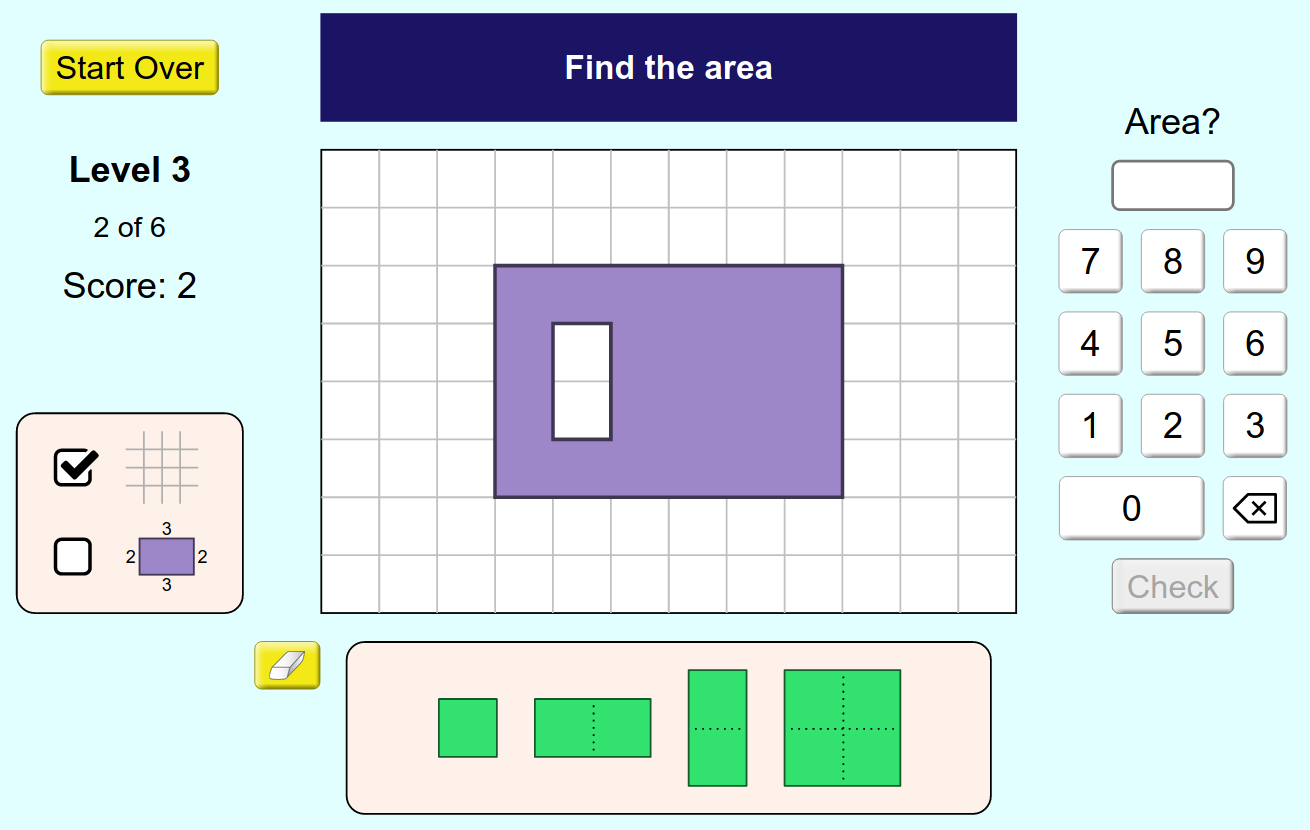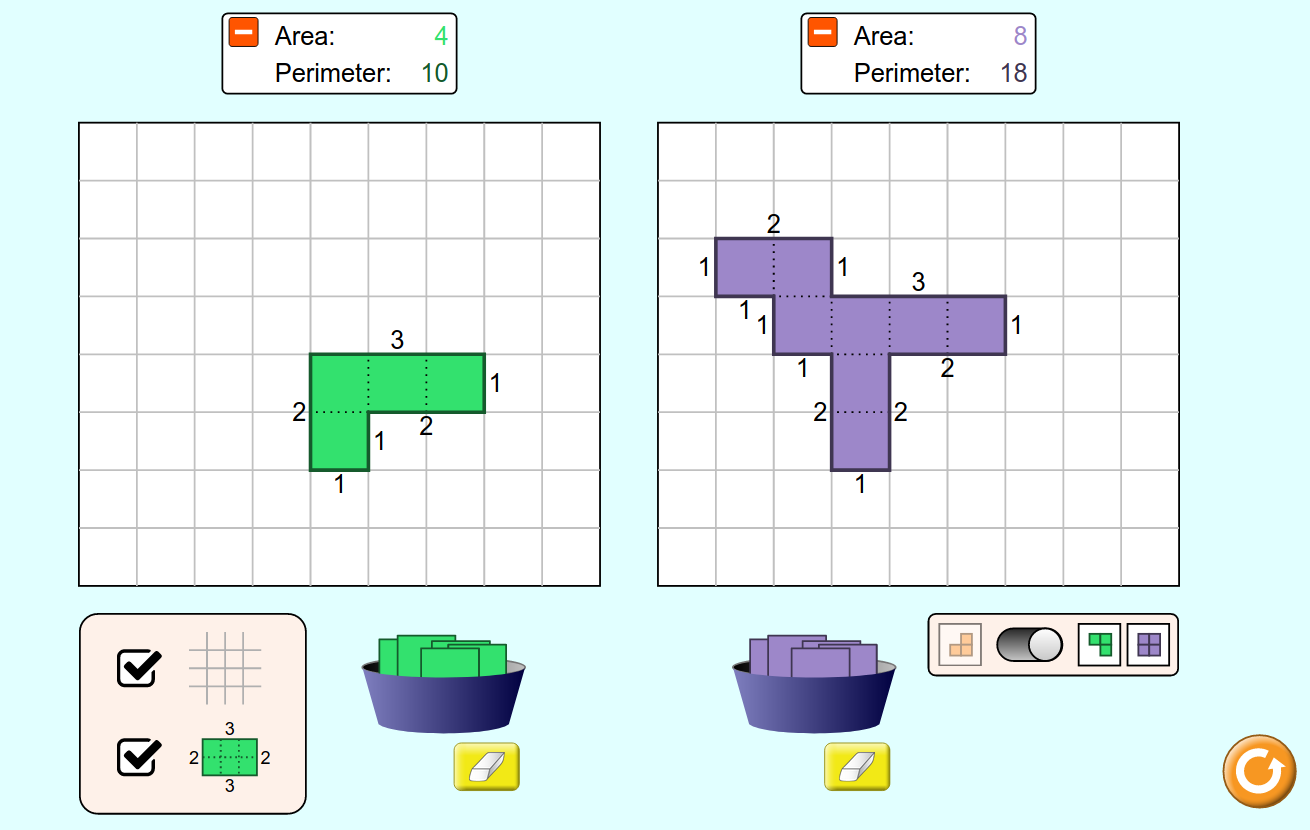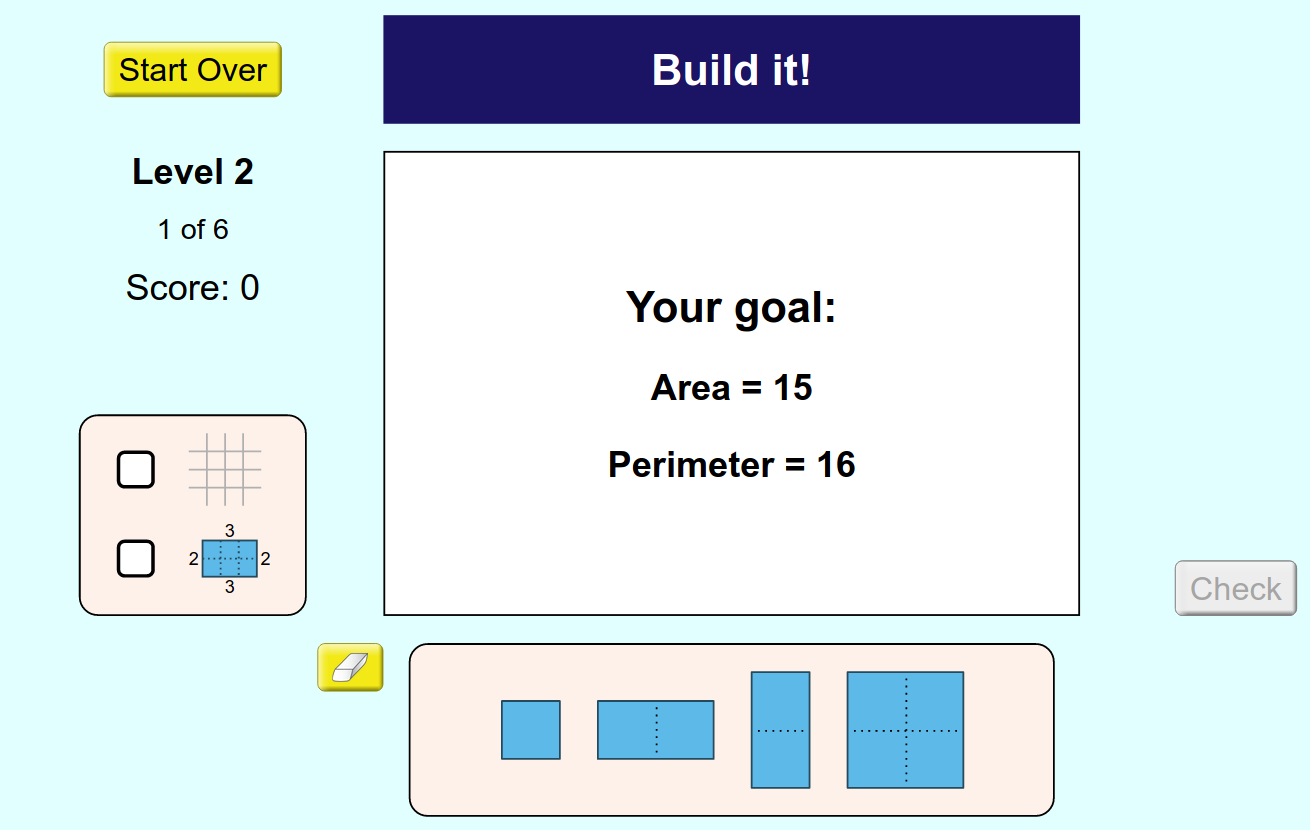MM Practice
×
Multiplication
Division
Place Value
Fractions & Decimals
Measurement
Statistics & Probability
Pre-Algebra
Money
Kindergarten
Geometry

# Area and Perimeter BuilderOnline activity and game for 2nd-4th grade math

This activity includes, first of all, a work area where you can explore area and perimeter, and then also a game.

In the work area, you can create your own shapes using colorful blocks, and explore the relationship between perimeter and area. You can also compare the area and perimeter of two shapes side-by-side.

In the game, you are either asked the area of a figure, or you are asked to BUILD a figure with a given area (and perimeter).

These activities will help students to find the area of a shape by counting unit squares, build shapes with a given area and/or perimeter, and find the area of an irregular shape by decomposing into smaller shapes (e.g., rectangles, triangles, squares).

Credit: This activity is created by PhET

Screenshots from the game and activity:Allow my comment to be posted on this site